# Mathematical Notation

1.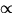- proportional to
2.
< or > - less than or greater than
3.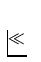or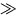- much less than or much greater than
4.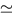- approximately equal to
5.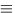- defined as
6.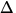x - change in the quantity x
7.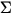- summation sign
8.
|x| - absolute value of x